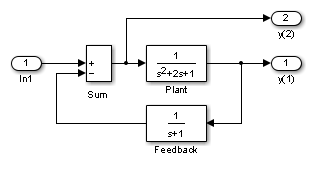The Simulink® trim function uses a model to determine steady-state points of a dynamic system that satisfy input, output, and state conditions that you specify. Consider, for example, this model, called ex_lmod.You can use the trim function to find the values of the input and the states that set both outputs to 1. First, make initial guesses for the state variables (x) and input values (u), then set the desired value for the output (y).

x = [0; 0; 0];
u = 0;
y = [1; 1];

Use index variables to indicate which variables are fixed and which can vary.

ix = [];      % Don't fix any of the states
iu = [];      % Don't fix the input
iy = [1;2];   % Fix both output 1 and output 2

Invoking trim returns the solution. Your results might differ because of roundoff error.

[x,u,y,dx] = trim('lmod',x,u,y,ix,iu,iy)

x =
0.0000
1.0000
1.0000
u =
2
y =
1.0000
1.0000
dx =
1.0e-015 *
-0.2220
-0.0227
0.3331

Note that there might be no solution to equilibrium point problems. If that is the case, trim returns a solution that minimizes the maximum deviation from the desired result after first trying to set the derivatives to zero. For a description of the trim syntax, see trim.